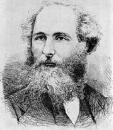Home

Lecture Notes

PHY 415: Electromagnetic Theory I
Prof. S. Teitel stte@pas.rochester.edu ---- Fall 2013

## Lecture Notes

The lecture note files correspond roughly to the material presented in a given day's lecture. But you may on occassion find the end of one day's lecture at the start of the file for the next day's lecture, so please look there if you think there might be something missing.

• Lecture 0 - A brief history of electromagnetism

• Lecture 1 - From Coulomb to Maxwell: charge, Coulomb's law, the electric field, differential and integral form of Maxwell's equations for electrostatics

• Lecture 2 - From Coulomb to Maxwell: Lorentz force, the magnetic field, Biot-Savart law, current density, charge conservation and the definition of magnetostatics, Maxwell's equations for magnetostatics

• Lecture 3 - Faraday's Law, Maxwell's correction to Ampere's Law, EM waves, systems of units, scalar and vector potentials, gauge invariance, Lorentz gauge

• Lecture 4 - Coulomb gauge, Longitudinal and transverse parts of a vector function, review of Fourier transforms

• Lecture 5 - Physical meaning of the electrostatic potential, Green's function, conductors in electrostatics, Coulomb problem as a boundary value problem, electric field at a charged surface, Dirichlet vs Neumann boundary condition

• Lecture 6 - Examples, Green's identities and uniqueness, the image charge method for a charge in front of an infinite plane
Green's functions, part II - Greens functions for Dirichlet and Neumann boundary conditions - we will not go over this in lecture.

• Lecture 7 - Image charges continued, charge in front of a conducting sphere

• Lecture 8 - Separation of variables method in rectangular and polar coordinates

• Lecture 9 - Separation of variables in spherical coordinates, Legendre polynomials

• Lecture 10 - Examples, multipole expansion
Green's functions, part III - Eigenfunction expansion for the Greens function - we did not go over this in lecture, but it provides some of the theoretical basis for why the separation of variables method works

• Lecture 11 - Multipole expansion, magnetostatics, magnetic dipole approximation

• Lecture 12 - Magnetic dipole approximation, magnetostatic scalar potential, boundary conditions at a sheet current, examples

• Lecture 13 - Example, symmetry under parity transformations, macroscopic Maxwell's equations

• Lecture 14 - Dielectrics, polarization density, electric displacement vector D, bound volume and surface charge density

• Lecture 15 - Macroscopic Maxwell's equations: magnetization density, H field, bound volume and surface current, vanishing of total bound charge and current

• Lecture 16 - Boundary conditions, linear materials: electric and magnetic susceptibilities, dielectric constant, magnetic permeability, atomic polarizability and the Clausius-Mossotti equation, screening of free charge

• Lecture 17 - Examples, point charge in a dielectric sphere

• Lecture 18 - Bar magnets, electromagnetism and conservation of energy, electromagnetic energy density, Poynting vector, conservation of momentum

• Lecture 19 - Maxwell stress tensor, force on a conducting surface, Capacitance matrix, inductance matrix, electromagnetic waves in a vacuum
Supplement - Force, torque, and interaction energy for electric and magnetic dipoles in an external field - we did not go over this in lecture.

• Lecture 20 - Electromagnetic waves in a vacuum, energy and momentum in electromagntic waves, frequency dependent atomic polarizability

• Lecture 21 - Frequency dependent dielectric function, electromagnetic waves in a dielectric: transparent propagation, resonant absorption, total reflection

• Lecture 22 - Electromagnetic waves in conductors: frequency dependent conductivity, low frequency "good" conductors, skin depth, high frequencies, longitudinal modes and plasma oscillations

• Lecture 23 - Linear, circular and elliptical polarization, waves at interfaces, angles of incidence, reflection and transmission, Snell's law, total internal reflection

• Lecture 24 - Snell's law for dissipative media, coefficient of reflection, total reflection, Brewster's angle

• Lecture 25 - Kramers-Kronig relation, Green's function for the wave equation, Lienard-Weichert potentials for a moving charged particle

• Lecture 26 - Potentials from a charged point particle moving with constant velocity, radiation from a source oscillating with simple harmonic motion, electric dipole, magnetic dipole, and electric quadrupole radiation

• Lecture 27 - Radiated E and B fields, Poynting vector and radiated power from an oscillating source

• Lecture 28 - Radiation from a general time dependent source, Larmor's formula for radiation from an accelerating charge, special relativity, Lorentz transformation, 4-vectors, proper time, 4-velocity, 4-gradient

• Lecture 29 - 4-current, 4-potential, field strength tensor and Maxwell's equations, energy-momentum 4-vector, Lorentz force, relativistic Larmor's formula